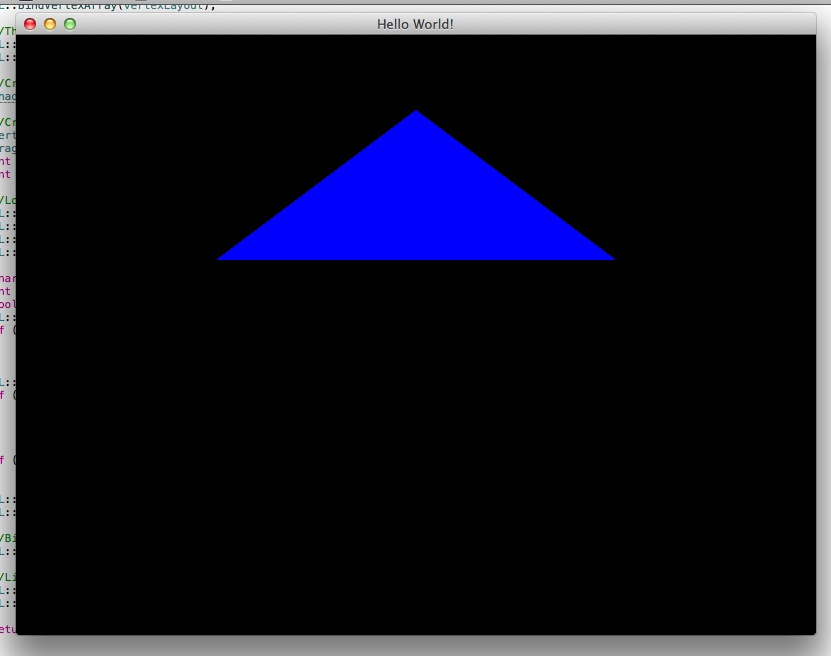# Modern Open GL Drawing a Triangle

So. Let’s talk about OpenGL. What a mess when you compare it with how DirectX has evolved over the years. I first learnt the intricacies of the DirectX API with the XNA framework which I used to create some simple games. This messing led me to start experimenting with shaders, ultimately leading to my work on Detour which had lots of fancy things like local point lights, realtime shadows, water reflection, particle systems and postprocessing effects.

One of the major problems with the DirectX API is that it only works on Windows. Microsoft no longer has the massive monopoly that it used to have and people expect games these days to run on every flavour of platform (Windows, Linux, OSX to name a few). So in practical terms this means you have to use OpenGL; an API that is supposed to work on every platform. What a brilliant idea! So.. how hard can it be to use OpenGL?

## Drawing a Triangle to the Screen

Lets try something simple, like drawing a triangle to the screen. Sounds good!

First we need to open a window. This part is platform specific, and people usually resort to a libraries like GLFW, GLEW and GLUT to handle things like creating a window, loading the OpenGL functions and handling mouse and keyboard input. For this post I’ll be using my own library I’ve cooked up called GLWT, the OpenGL Window Toolkit! The toolkit is very much a work in progress but enough stuff is working for me to be able to write this post. It only works for Macs at the moment, but I plan to make it work on Windows and Linux eventually.

Next we need to think about which version of the OpenGL API to use. OpenGL has a long history and a lot of old applications use the old, immediate mode API. These are the functions that pop up everywhere whenever you type ‘OpenGL tutorial’ into Google. For example, this is the old way of drawing a triangle to a screen:

``````void Draw()
{
glBegin(GL_TRIANGLE_STRIP);
glVertex2f(0.0f, 0.75f);
glVertex2f(-0.5f, 0.25f);
glVertex2f(0.5f, 0.25f);
glEnd();
}
``````

The code above, whilst simple, is innefficient since you have to copy the triangles from the CPU to GPU every single frame. You also get loads of function calls and User -> Kernel mode switches. You can’t do cool things with this setup like vertex and pixel/fragment shaders, or geometry shaders, or render to a texture, or add realtime shadows, etc. Ouch.

So what’s the solution? OpenGL Core Profile! This removes all of the old fixed pipeline calls and replaces them with buffer objects which you only update when you need to. It is a lot more complicated, but you can do way more cool stuff. Here’s how you draw a triangle to the screen:

``````struct Vertex
{
float x, y, z;
};

GLuint vertexBuffer, indexBuffer, vertexLayout;

bool Game::Setup(int argc, const char** argv)
{
Window::Open(800, 600, false, "Hello World!");

GL::ClearColor(0.0f, 0.0f, 0.0f, 1.0f);

Vertex verts[] =
{
{ 0.0f, 0.75f, 0.0f },
{ -0.5f, 0.25f, 0.0f },
{ 0.5f, 0.25f, 0.0f }
};
unsigned short inds[] =
{
0, 1, 2
};

//Create the vertex buffer object, then set it as the current buffer, then copy the vertex data onto it.
GL::GenBuffers(1, &vertexBuffer);
GL::BindBuffer(GL_ARRAY_BUFFER, vertexBuffer);
GL::BufferData(GL_ARRAY_BUFFER, sizeof(verts), &verts, GL_STATIC_DRAW);

//Create the index buffer object, set it as the current index buffer, then copy index data to it.
GL::GenBuffers(1, &indexBuffer);
GL::BindBuffer(GL_ELEMENT_ARRAY_BUFFER, indexBuffer);
GL::BufferData(GL_ELEMENT_ARRAY_BUFFER, sizeof(inds), &inds, GL_STATIC_DRAW);

//Create the vertex layout
GL::GenVertexArrays(1, &vertexLayout);
GL::BindVertexArray(vertexLayout);

//The vertex layout has 3 floats for position
GL::VertexAttribPointer(0, 3, GL_FLOAT, GL_FALSE, sizeof(Vertex), BUFFER_OFFSET(0));
GL::EnableVertexAttribArray(0);

return true;
}

void Game::Draw(float deltaTime)
{
GL::Clear(GL_COLOR_BUFFER_BIT | GL_DEPTH_BUFFER_BIT);

GL::BindBuffer(GL_ELEMENT_ARRAY_BUFFER, indexBuffer);
GL::BindBuffer(GL_ARRAY_BUFFER, vertexBuffer);
GL::DrawRangeElements(GL_TRIANGLES, 0, 3, 3, GL_UNSIGNED_SHORT, NULL);
}
``````

As you can see this is much more complicated, however the code above is not enough for the triangle to appear on the screen. We need to write a vertex shader and a fragment shader first. Blimey. Lets see how to do that.

OpenGL shaders are written in a language called GLSL, the OpenGL Shading Language.

Vertex shaders are basically a function that gets executed by your graphics card for every vertex, and it is usually where you do things like multiply it by a world view projection matrix to get the 2D position on the screen. Here is an example which just returns the vertex position without doing any processing:

``````in vec3 inVertex;
void main()
{
gl_Position = vec4(inVertex, 1.0);
}
``````

Fragment shaders are a function that gives the colour for each pixel in the triangle being drawn. The example shown here gives the triangle a nice blue colour. Yum:

``````out vec4 FragColor;
void main()
{
FragColor = vec4(0.0, 0.0, 1.0, 1.0);
}
``````

## Putting it all Together

So we now have some GLSL code that will put the triangles onto the screen in a nice blue colour. We now need to tell OpenGL to load all of that shader code, turn it into a single shader object and apply it to the triangle we will draw to the screen. This code will load and compile the vertex and fragment shader, then put them into a shader program object:

``````//Create the shader program

//Create the vertex and fragment shader

char log;
int len;
if (len > 0) {
std::cout << "Vertex Compile error:" << std::endl << log << std::endl;
}
if (len > 0) {
std::cout << "Fragment Compile error:" << std::endl << log << std::endl;
}

return false;

//Bind the vertex position to the inVertex variable in the vertex shader